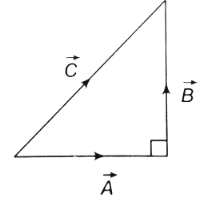## Physics MCQ on Addition and subtraction of Vector for NEET, JEE, Medical and Engineering Exam 2022

MCQ on Addition and subtraction of Vector: A vector quantity is defined as the physical quantity that has both direction as well as magnitude. A vector with the value of magnitude equal to one and direction is called unit vector represented by a lowercase alphabet with a “hat” circumflex. That is “û“.

In this post we are providing you MCQ on Addition and subtraction of Vector, which will be beneficial for you in upcoming NEET, AIIMS, JEE, WBJEE, Medical and Engineering Exams.## MCQ on Addition and subtraction of Vector

Q1. If the magnitude of the resultant force of two forces is lesser than the magnitude of the larger force, then
a) The two forces are perpendicular to each other
b) They two forces in opposite direction
c) The forces are different in magnitude and direction
d) The forces posses small magnitude

(b) They two forces in opposite direction

Q2. If |A-B| = |A| = |B|, then what is the angle between A and B?
a) 0°
b) 30°
c) 60°
d) 90°

(c)60°

Q3. Two  forces F1 and F2 that are perpendicular to each other act on a point mass. The resultant force in the point mass is given by
a) F1+F2
b) F1-F2
c) F1²+F2²
d) √F1²+F2²

Q4. If  three vectors A, B and C have magnitudes 5, 12 and 13 and A⃗ +B⃗ =C⃗ , then what is the angle between B and C?
a)
b)
c)
d)

Q5. Which of the following is true for the given diagram?a) A+B= C
b) C+A = B
c) B+C = A                                                                                                                                                                        d) A+B+C=0

(B) C+A=B

Q6. A person travels 10 km North and 20 km East. What will be the displacement from the initial point?
a) 20 km
b) 22.36 km
c) 30 km
d) 32.36 km

(b) 22.36 km

Q7.If the sum of two unit vectors is 1,then what is the difference between them?
a) 1/√2
b) √2
c) √3
d) √4

(c) √3

Q8. Two  forces F1 and F2 act on a body. One of the forces is double of the other force. The resultant of these forces is equal to the larger force. What is the angle between the forces?
a) cos1(1/2)
b) cos1(-1/2)
c) cos1(1/4)
d) cos1(-1/4)

d) cos1(-1/4)

Q9.At What angle should the two force vectors 5N and 12N be added to get a resultant vector of 13N?
a) 00
b) 300
c) 900
d) 1800

(c) 900

Q10.What is the net force on the particle if it is simultaneously acted upon by two forces 4 N and 3 N?
a) 1 N
b) 7 N
c) 21 N
d) Between 7 N and 1 N

(d) Between 7 N and 1 N